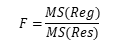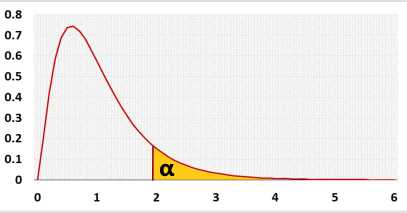# Multiple Linear Regression Calculator

Multiple regression calculator with unlimited predictors.

Constant is zero (Force zero Y-intercept, b0=0)
Power regression - Ln transformation (natural log) over all the variables: Y=exp(b0)⋅X1b1⋅⋅Xpbp .
Enter raw data directly
Enter raw data from excel

Header: You may change the groups' names to their real names.
Data: When entering data, press Enter or Comma , or Space after each value.
* All variables will be included in the automatic iterations mode.
** Normality colors based on α=0.05

## Multiple linear regression calculator

The calculator uses variables transformations, calculates the Linear equation, R, p-value, outliers and the adjusted Fisher-Pearson coefficient of skewness.
After checking the residuals' normality, multicollinearity, homoscedasticity and priori power, the program interprets the results.
Then, it draws a histogram, a residuals QQ-plot, a correlation matrix, a residuals x-plot and a distribution chart.
You may transform the variables, exclude any predictor or run backward stepwise selection automatically based on the predictor's p-value.

Right-tailed F test. Checks if the entire regression model is statistically significant. Why?

## Multiple linear regression formula

Y = b0 + b1X1 + b2X2 + b3X3+...+ bpXp + ε
It is easier to use the matrix form for multiple linear regression calculations:
Y = XB + Ε
Ŷ = XB
B = (X'X)-1X'Y
 [1 X11 X12 ... X1p] [Y1] ε1] [1 X21 X22 ... X2p] [Y2] [ε2] X = [1 X31 X32 ... X3p] Y = [Y3] Ε = [ε3] [1 X41 X42 ... X4p] [Y4] [ε4] [1 Xn1 Xn2 ... Xnp] [Yn] [εn]
 [B0] [B1] B = [B2] [... ] [Bp]
Y - dependent variable vector.
Ŷ - predicted Y vector.
Ε - residuals vector, Ε = Y - Ŷ.
p - number of predictors.
n - sample size.
Hypotheses
H0: Y = b0
H1: Y=b0+b1X1+...+bpXp
Test statisticF distribution